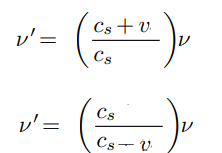# Changing of frequencies in the Doppler effect

• LCSphysicist

#### LCSphysicist

Homework Statement
All below
Relevant Equations
All below
I am trying to figure out why the relativity don't get in the doppler effect, that is, why can't we apply the "galileo transformations" in doppler effect?
OBS: i am not saying about doppler effect relativistic (using Lorentz transformation)

In another words, i am moving in direction to a source with velocity v, we use the first case below.
But if i imagine that actually the font is going in my direction, as classical mechanics allows, i could try to use the second case.I'm not entirely sure what you're trying to do, but the non-relativistic Doppler effect can be derived from the Galilean transformation. For instance, let us consider a stationary source. Take a wave traveling in the ##-\hat{x}## direction in the rest frame of the medium, $$y = A\sin{(kx + \omega t)}$$Now let us transform into a frame moving at ##v_x = V##, such that ##x' = x - Vt## (and ##t = t'##), then the equation of the wave is$$y' = A\sin{(kx' + (kV + \omega)t')}$$You can see that ##\omega' = kV + \omega## and ##k' = k##. These imply that ##\lambda = \lambda'## and ##f' = \frac{V}{\lambda} + f = \left(\frac{V}{c} + 1 \right)f = \left (\frac{V+c}{c} \right)f##, which is the first formula you write.

Last edited by a moderator:
Homework Statement:: All below
Relevant Equations:: All below

I am trying to figure out why the relativity don't get in the doppler effect, that is, why can't we apply the "galileo transformations" in doppler effect?
OBS: i am not saying about doppler effect relativistic (using Lorentz transformation)

In another words, i am moving in direction to a source with velocity v, we use the first case below.
But if i imagine that actually the font is going in my direction, as classical mechanics allows, i could try to use the second case.

View attachment 267525
You can. But you must consider that in the second case, the medium itself is moving. So in the second case, you'll need to first do a Galilean transformation such that $v$ represents a velocity relative to the medium. Once you do that, the first equation pops back out.

In other words, in the more general equations of $\nu' = \left( \frac{c_s \pm v_r}{c_s \pm v_s} \right) \nu$, where $v_r$ is the speed of the receiver and $v_s$ is the speed of the source, it is assumed that these velocities are measured with respect to the velocity of the medium itself.

(Of course, as already mentioned, this applies to things that have a medium, such as sound. The above does not apply to light, electromagnetic waves, and/or Einstein's relativity.)

•etotheipi
Under a Galilean shift to a frame traveling at ##v##, the speeds of the observer, source, and wave are ##v_o' = 0##, ##v_s' = v## and ##c' = c+v## respectively (be careful with signs when dealing with boosted speeds). Use your second formula to write$$f' = \left( \frac{c'}{c'-v_s'} \right)f = \left( \frac{c + v}{c} \right)f$$i.e. we recover the first equation, as @collinsmark points out. That is to say we just measure the speeds of the observer, source and wave w.r.t. our frame - where wave speed equals the vector sum of medium speed and relative speed of the wave w.r.t. the medium.

Last edited by a moderator: